Web www.handwritingforkids.com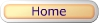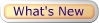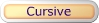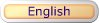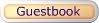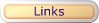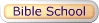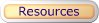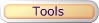### Online Math 1 - Multiplication Math Drill/Test Multiplication by Factors (Vertical)

This online/interactive generator will provides plenty of math drill and practice in multiplication, to boost test scores, improves accuracy and speed to prepare the child/children to meet the Grades 3-5 curriculum standards. This online/interactive math multiplication, will create 1 digit by 1 digit (1x1) equations with an option of timed test, and it will automatically scored your results in a printable worksheet.

Type of online math problems:

• Multiplication - Online Math 1 - Factor of Three (3)
• Multiplication - Online Math 1 - Factor of Six (6)
• Multiplication - Online Math 1 - Factors Zero to Four
• Multiplication - Online Math 1 - Factors Zero to Eight
• and many more!

 3 x 3 9factorfactorproduct

2. Select the number of math problems per page. (Default: 1 - 20)
3. Select Type of Math Problems. (Default: Difference 0 to 10)
4. Do you want a timed-test? (Default: Yes)
5. Click on submit to make your personalized online vertical math multiplication drill/test.
6. Option: Page back and click Submit to create a different set of math problems.

Online Math 1 - Multiplication Math Drill/Test Maker (Vertical)

 Name: (optional)

Enter the Number of Math Problems per page:

Default: 1 - 20

Type of Math Problems:

Default: factors to nine

Yes, Timed-Test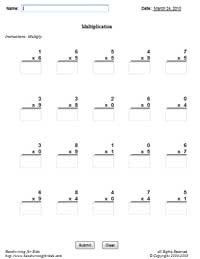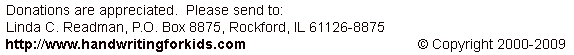Basic Handwriting for Kids Free for Non-Profit Use Ms. Readman, Rockford, IL Revised: